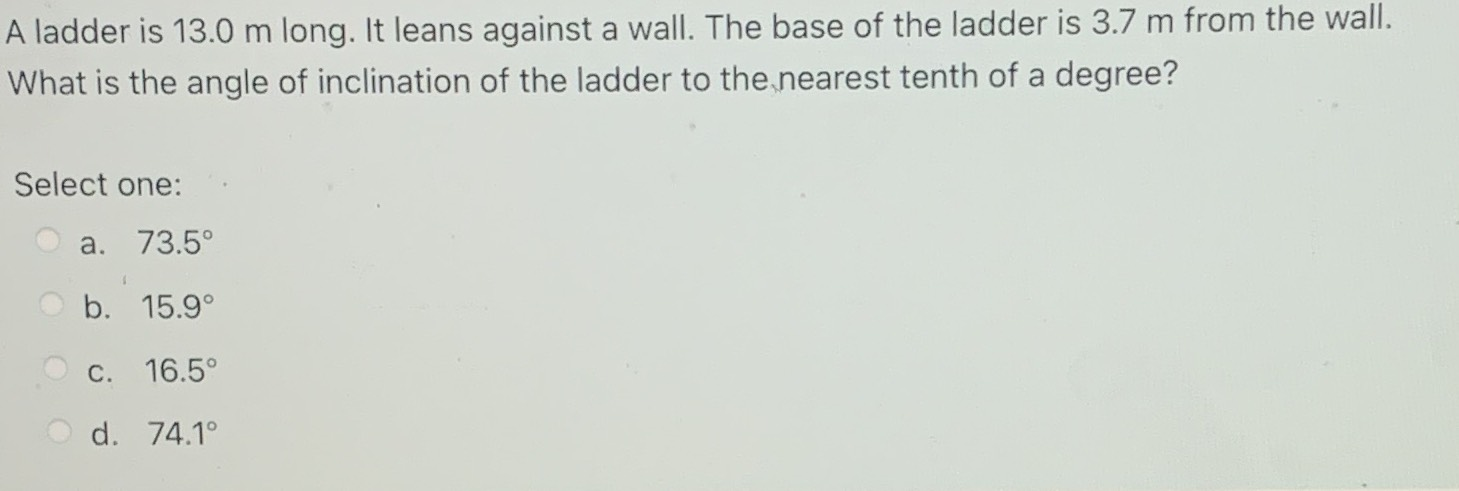### Still have math questions?

Trigonometry
QuestionA ladder is $$13.0$$ m long. It leans against a wall. The base of the ladder is $$3.7 m$$ from the wall. What is the angle of inclination of the ladder to the nearest tenth of a degree? Select one:

a. $$73.5 ^ { \circ }$$

b. $$15.9 ^ { \circ }$$

c. $$16.5 ^ { \circ }$$

d. $$74.1 ^ { \circ }$$